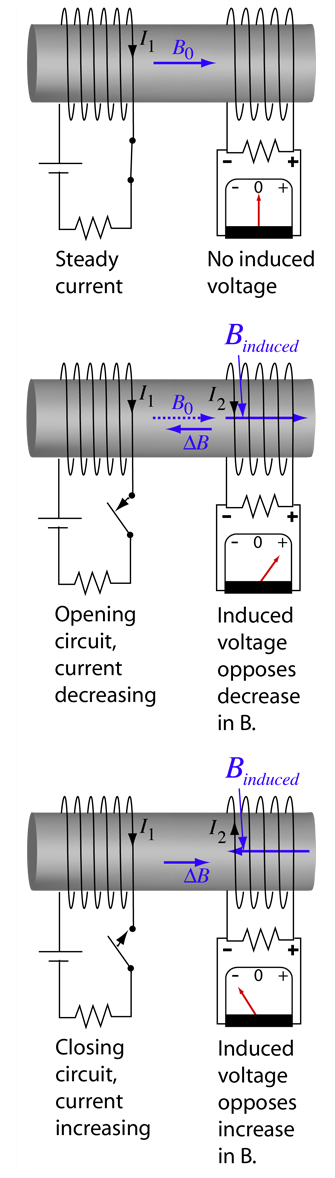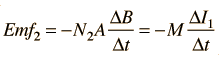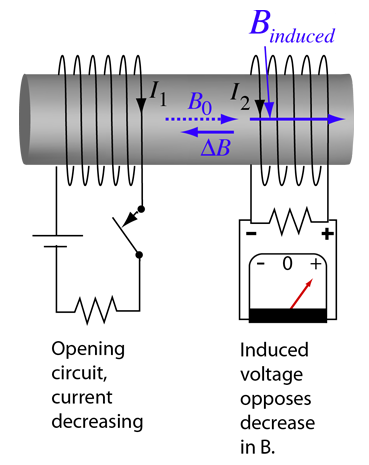# Coupled Inductors

 When a steady current flows in one coil as in the left illustration, a magnetic field is produced in the other coil. But since that magnetic field is not changing, Faraday's law tells us that there will be no induced voltage in the secondary coil. But if the switch is opened to stop the current as in the middle illustration, there will be a change in magnetic field in the right hand coil and a voltage will be induced. A coil is a reactionary device, not liking any change! The induced voltage will cause a current to flow in the secondary coil which tries to maintain the magnetic field which was there. The fact that the induced field always opposes the change is an example of Lenz' law. Once the current is interrupted and the switch is closed to cause the current to flow again as in the right hand example, an induced current in the opposite direction will oppose that buildup of magnetic field. This persistent generation of voltages which oppose the change in magnetic field is the operating principle of a transformer. The fact that a change in the current of one coil affects the current and voltage in the second coil is quantified in the property called mutual inductance.Index

Inductance concepts

 HyperPhysics***** Electricity and Magnetism R Nave
Go Back

# Mutual Inductance

 When an emf is produced in a coil because of the change in current in a coupled coil , the effect is called mutual inductance. The emf is described by Faraday's law and it's direction is always opposed the change in the magnetic field produced in it by the coupled coil (Lenz's law ). The induced emf in coil 1 is due to self inductance L. The induced emf in coil #2 caused by the change in current I1 can be expressed asThe mutual inductance M can be defined as the proportionalitiy between the emf generated in coil 2 to the change in current in coil 1 which produced it.

The most common application of mutual inductance is the transformer.

 Coil's reaction to increasing current
Index

Inductance concepts

AC Circuits

 HyperPhysics***** Electricity and Magnetism R Nave
Go Back

# Mutual Inductance:Transformer

When more current flows in the secondary of a transformer as it supplies more power, then more current must flow in the primary as well since it is supplying the energy. This coupling between the primary and secondary is most conveniently described in terms of mutual inductance. The mutual inductance appears in the circuit equations for both the primary and secondary circuits of the transformer.Calculation
Index

Inductance concepts

Transformer concepts

AC Circuits

 HyperPhysics***** Electricity and Magnetism R Nave
Go Back